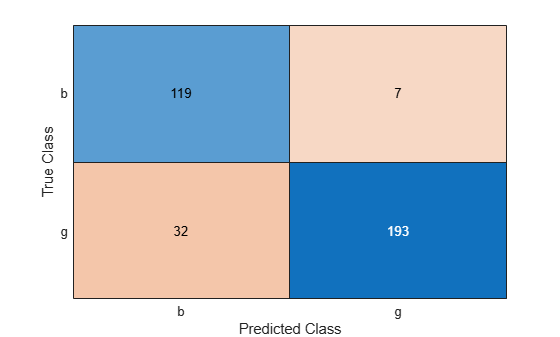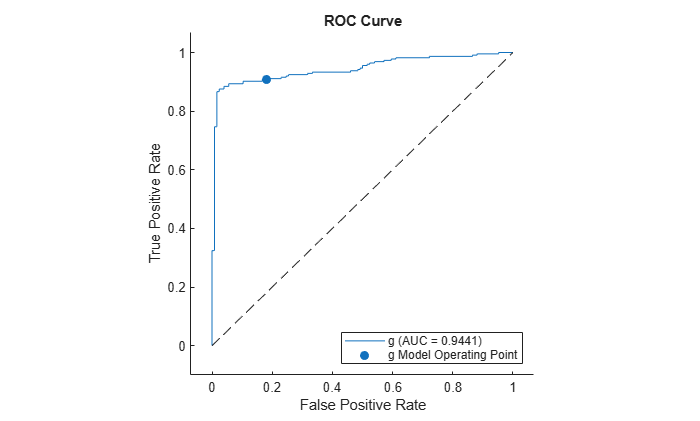# kfoldPredict

Classify observations in cross-validated kernel classification model

## Syntax

``label = kfoldPredict(CVMdl)``
``[label,score] = kfoldPredict(CVMdl)``

## Description

example

````label = kfoldPredict(CVMdl)` returns class labels predicted by the cross-validated, binary kernel model (`ClassificationPartitionedKernel`) `CVMdl`. For every fold, `kfoldPredict` predicts class labels for validation-fold observations using a model trained on training-fold observations.```

example

````[label,score] = kfoldPredict(CVMdl)` also returns classification scores for both classes.```

## Examples

collapse all

Classify observations using a cross-validated, binary kernel classifier, and display the confusion matrix for the resulting classification.

Load the `ionosphere` data set. This data set has 34 predictors and 351 binary responses for radar returns, which are labeled either bad (`'b'`) or good (`'g'`).

`load ionosphere`

Cross-validate a binary kernel classification model using the data.

```rng(1); % For reproducibility CVMdl = fitckernel(X,Y,'Crossval','on')```
```CVMdl = ClassificationPartitionedKernel CrossValidatedModel: 'Kernel' ResponseName: 'Y' NumObservations: 351 KFold: 10 Partition: [1x1 cvpartition] ClassNames: {'b' 'g'} ScoreTransform: 'none' Properties, Methods ```

`CVMdl` is a `ClassificationPartitionedKernel` model. By default, the software implements 10-fold cross-validation. To specify a different number of folds, use the `'KFold'` name-value pair argument instead of `'Crossval'`.

Classify the observations that `fitckernel` does not use in training the folds.

`label = kfoldPredict(CVMdl);`

Construct a confusion matrix to compare the true classes of the observations to their predicted labels.

`C = confusionchart(Y,label);`The `CVMdl` model misclassifies 32 good (`'g'`) radar returns as being bad (`'b'`) and misclassifies 7 bad radar returns as being good.

Estimate posterior class probabilities using a cross-validated, binary kernel classifier, and determine the quality of the model by plotting a receiver operating characteristic (ROC) curve. Cross-validated kernel classification models return posterior probabilities for logistic regression learners only.

Load the `ionosphere` data set. This data set has 34 predictors and 351 binary responses for radar returns, which are labeled either bad (`'b'`) or good (`'g'`).

`load ionosphere`

Cross-validate a binary kernel classification model using the data. Specify the class order, and fit logistic regression learners.

```rng(1); % For reproducibility CVMdl = fitckernel(X,Y,'Crossval','on', ... 'ClassNames',{'b','g'},'Learner','logistic')```
```CVMdl = ClassificationPartitionedKernel CrossValidatedModel: 'Kernel' ResponseName: 'Y' NumObservations: 351 KFold: 10 Partition: [1x1 cvpartition] ClassNames: {'b' 'g'} ScoreTransform: 'none' Properties, Methods ```

`CVMdl` is a `ClassificationPartitionedKernel` model. By default, the software implements 10-fold cross-validation. To specify a different number of folds, use the `'KFold'` name-value pair argument instead of `'Crossval'`.

Predict the posterior class probabilities for the observations that `fitckernel` does not use in training the folds.

`[~,posterior] = kfoldPredict(CVMdl);`

The output `posterior` is a matrix with two columns and `n` rows, where `n` is the number of observations. Column `i` contains posterior probabilities of `CVMdl.ClassNames(i)` given a particular observation.

Obtain false and true positive rates, and estimate the area under the curve (AUC). Specify that the second class is the positive class.

```[fpr,tpr,~,auc] = perfcurve(Y,posterior(:,2),CVMdl.ClassNames(2)); auc```
```auc = 0.9441 ```

The AUC is close to `1`, which indicates that the model predicts labels well.

Plot an ROC curve.

```plot(fpr,tpr) xlabel('False positive rate') ylabel('True positive rate') title('ROC Curve')```## Input Arguments

collapse all

Cross-validated, binary kernel classification model, specified as a `ClassificationPartitionedKernel` model object. You can create a `ClassificationPartitionedKernel` model by using `fitckernel` and specifying any one of the cross-validation name-value pair arguments.

To obtain estimates, `kfoldPredict` applies the same data used to cross-validate the kernel classification model (`X` and `Y`).

## Output Arguments

collapse all

Predicted class labels, returned as a categorical or character array, logical or numeric matrix, or cell array of character vectors.

`label` has n rows, where n is the number of observations in `X`, and has the same data type as the observed class labels (`Y`) used to train `CVMdl`. (The software treats string arrays as cell arrays of character vectors.)

`kfoldPredict` classifies observations into the class yielding the highest score.

Classification scores, returned as an n-by-2 numeric array, where n is the number of observations in `X`. `score(i,j)` is the score for classifying observation `i` into class `j`. The order of the classes is stored in `CVMdl.ClassNames`.

If `CVMdl.Trained{1}.Learner` is `'logistic'`, then classification scores are posterior probabilities.

collapse all

### Classification Score

For kernel classification models, the raw classification score for classifying the observation x, a row vector, into the positive class is defined by

`$f\left(x\right)=T\left(x\right)\beta +b.$`

• $T\left(·\right)$ is a transformation of an observation for feature expansion.

• β is the estimated column vector of coefficients.

• b is the estimated scalar bias.

The raw classification score for classifying x into the negative class is f(x). The software classifies observations into the class that yields a positive score.

If the kernel classification model consists of logistic regression learners, then the software applies the `'logit'` score transformation to the raw classification scores (see `ScoreTransform`).